# Where is the electron in an orbital

### Orbitals

Now we want to apply the Schrödinger wave mechanics to the Electrons in the atomic shell apply. In contrast to the force-free movement of an electron in a potential well, the electrostatic attraction of the positively charged nucleus acts on the electrons in an atom. The potential energy of the electrons is no longer zero. Therefore the Schrödinger equation is more complicated for an electron in an atomic lattice than for a free electron in a potential well:

 Three-dimensional, time-independent Schrödinger equation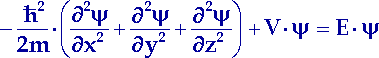(13.1)

The potential energy of the electron only depends on its radial distance r to the atomic nucleus (Z: atomic number):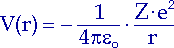(13.2)

That is why it makes sense to use spherical coordinates instead of Cartesian coordinates: so-called Polar coordinates, where a point in space by specifying its distance r from the center point and two angles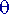andis established between the vector r and spatial directions of the Cartesian coordinates. We can see the relationship between polar and Cartesian coordinates in the figure on the right. The distance r then results in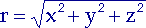(13.3)

The conversion of the Schrödinger equation into polar coordinates requires knowledge of the differentiation rules for functions of several variables. The result of a rather lengthy calculation is the following equation:(13.4)

To solve a differential equation of this kind, the three variables r,andseparated by writing the wave function as the product of functions of the individual variables:(13.5)

Let's put this one Separation approach For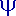into equation (13.4), then we can obtain three ordinary differential equations, each with only one variable: one differential equation each for the radial component R (r) and the angular components() and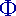(). However, these three equations are characterized by three constants, the so-called Separation constants, or Quantum numbersn, m and l connected with each other.

In contrast to quantum numbers for an electron in the three-dimensional potential well, the numbers n, m and l depend on each other, in the following way:

 n = 1, 2, 3, ...l = 0, 1, 2, ..., (n - 1)m = -l, - (l - 1), ..., 0, ..., + (l - 1), + l (13.6)

According to this, n is any natural number, l is an integer between 0 and n - 1, and m takes on integer values ​​from - 1 to + 1.

The number n becomes Principal quantum number called. It is related to the radial component of the wave function R (r) and thus corresponds roughly to the number n in Bohr's atomic model. The main quantum number denotes the Bowlto which an electron belongs.

In contrast to Bohr's atomic model, a shell in wave mechanics is not a defined circular path, but an area in which the probability of an electron being located is relatively high.

The larger n is, the further away the shell is from the atomic nucleus and the higher the energy of the electron. The shells are often referred to as Bohr orbits with capital letters K, L, M, N, etc. Is z. B. the electron of the hydrogen atom in the K-shell (n = 1), then the atom is in Basic state: its lowest energy state.

In the case of hydrogen, the energy of the electron depends only on the principal quantum number. This is why its spectrum can be perfectly explained using Bohr's atomic model. However, spectral studies of atoms with several electrons show more complicated line spectra. If an atom has several electrons, they interact with each other and an additional electric field is built up. The potential energy of electrons no longer depends exclusively on their distance from the nucleus, i.e. on the principal quantum number, but can also assume different values ​​in the same shell. Electrons of a shell can therefore be in different, but adjacent energy levels - Lower shells - stop. Each lower shell comes with a Minor quantum numberlor designated with a letter:

 l =Symbol: 0, 1, 2, 3, 4, ... s, p, d, f, g, ... (13.7)

The first four letters come from spectroscopy, some spectral lines used to be with English adjectives sharp, principal, diffuse, fundamental named. From l> 3 the letters are continued alphabetically. This gives each subshell a simple and concise name: The subshell with n = 1 and l = 0 is called 1s; 2p stands for the subshell with n = 2 and l = 1. The energy of a subshell is determined by two quantum numbers: n and l. Within a shell, the energy of the electrons increases with an increasing secondary quantum number, i.e. in the order: s

How can we visualize the distribution of electrons in a subshell?

A subshell is determined by the quantum numbers n and l. Therefore we need that part of the wave function in which only these two quantum numbers occur, namely the radial function R (r). The general form of a normalized radial function looks like this: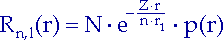(13.8)

Where r1 the first Bohr radius, N a normalization factor and p (r) a polynomial in r.

According to Born's statistical interpretation, the probability is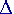P that an electron in a volumeV comes into effect, equal to the product of the square of the magnitude of its normalized wave function andV: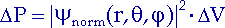(13.9)

The distribution function of electrons in a subshell may only depend on one variable if we want to represent it in two dimensions. Therefore we replace the normalized wave function in (13.9)against its radial part R (r). Since R (r) is a real-valued function, it can itself be squared. AsV we take a thin spherical shell, its volume is equal to the product of the surface of the shell 4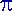r2 and their thicknessr. ThenP (r) is the radial probability of an electron: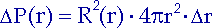(13.10)

and his radial probability density,P (r), the distribution function we are looking for:(13.11)

In the diagram on the left we see the radial probability densities for the subshells 1s, 2s and 2p in a hydrogen atom. We see that an electron of the 1s subshell has its greatest probability of being at the first Bohr radius. If an electron is in the 2s subshell, then P (r) has two maxima. The largest maximum of 2s and the maximum of 2p lie near the second Bohr radius (4r1). The zero of P (r) for 2s means that the probability of a 2s electron being in a spherical shell around the nucleus with the radius 2r1 is almost zero. Such spherical surfaces are Nodal spherical surfaces called.

The quantum number l also appears in the angle-dependent part of the wave function(). It determines the amount of the Orbital angular momentumL =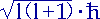, which is calculated from the Schrödinger equation. The minor quantum number l is therefore also called Orbital angular momentum quantum number. Analogous to the angular momentum of a classical particle, we can think: An electron revolves around an atomic nucleus, its orbital angular momentum vector L is perpendicular to the plane of rotation. The electron thus represents an electric current and consequently generates a magnetic field. If we bring an atom into a magnetic field, this external field tries to turn the levels of the electron orbits (just like the current loops of an electric motor). Depending on the orientation of the planes of rotation in space, the directions of the angular momentum vectors change and thus also the energy of the electrons.

We can see this phenomenon in the splitting of certain spectral lines (Zeeman effect) observe. An electron transfer from one subshell to another corresponds to a line in the spectrum without a magnetic field. In addition to this line, other higher and lower lying lines appear in the magnetic field. The energy of the lower shell splits into a total of 2l + 1 levels. Thus 2l + 1 certain orientations of the orbital angular momentum vector to the magnetic field are detected. Each of these orientations comes with a Magnetic quantum number or Orientation quantum number m (from - l to + l). The picture on the left shows the permitted orientations of the angular momentum for l = 1. If the magnetic field points in the z-direction, then the following applies to the z-component of the orbital angular momentum: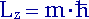. Because of the uncertainty relation, we can only specify one component of the angular momentum exactly. The x and y components are indeterminate. The vectors lie at undefined points on the cone surfaces around the z-axis and on the circle around the origin.

In the absence of a magnetic field, the electrons in a subshell cannot be distinguished from one another. All directions of the orbital angular momentum vector are energetically equivalent. That is why the electrons in a subshell have the same energy. By applying a magnetic field, we determine: electrons of a subshell can be in 2l + 1 different quantum states, each of these states corresponds to a certain wave function.

The wave functions of electrons in an atom are called atomic orbitals. The atomic orbitals describe the spatial distribution of the probability of an electron being located. Each orbital is represented by three quantum numbers n, l and m clearly marked. The number of orbitals in a subshell l is equal to 2l + 1. The magnetic quantum number is used to distinguish the orbitals of a subshell. All orbitals of a subshell have the same energy, they are degenerate.

How can we visualize the distribution of electrons in atomic orbitals?

Atomic orbitals are graphically mostly represented by contour areas of the electron density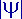2, so-called Interface diagrams, shown. These are areas of constant value of2. They limit areas of space in which an electron is with a certain probability (e.g. 90%):

In the figure above we can see that s orbitals have a spherical distribution of electron density. In the case of p orbitals, two areas of great probability are separated by a nodal plane that runs through the atomic nucleus. Because of its rotational symmetry, the image of a p orbital appears like a dumbbell with two spheres on either side of the nodal plane. In order to distinguish the three orbitals of a p-subshell, they are drawn rotationally symmetrical with respect to an axis of the coordinate system and according to their rotational axes px-, py- or pzCalled the orbital. The interface diagrams of d orbitals have two nodal levels. You own (apart from the dz2-Orbital) has a rosette-shaped shape. The secondary quantum number l indicates the number of nodal levels and thus determines the shape of the orbitals. Knowledge of the orbital structure of atoms and molecules is necessary to understand chemical bonds.